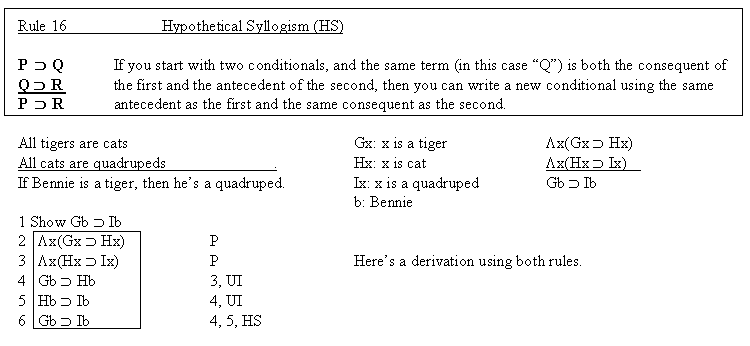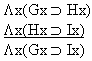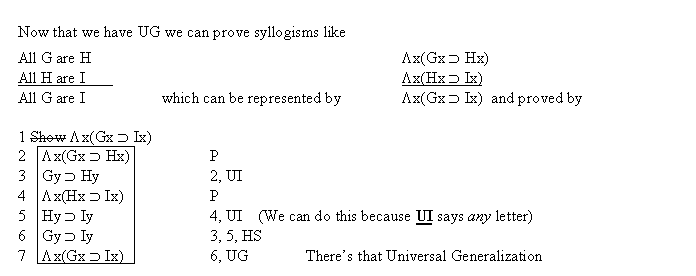Logic Chapter Twenty Two
Next Chapter

Here's a fairly simple, easy to understand rule. It works very well with Universal Instantiation.But what if we want to prove something likeWell, we need a rule like the following:Hints: Can names be replaced? What about bound variables? How many instances must be replaced? Does UG use "∃?"
(Remember, a name is a lower case letter from "a" to "t," a variable is a lower case letter from "w" to "z"
In logic, a syllogism is a three line argument in which all three lines (two premises and a conclusion) are universal statements. Aristotle, who founded formal logic, studied syllogisms intensively and came up with all kinds of rules and special drawings to explain the relationships between different types of universal statements, and to determine the validity of various types of syllogisms. I don't teach this stuff because it's very difficult, very boring, and the logic of it all can be communicated effectively without Aristotle's terminology.Quiz preparation: Mark the following true or false based on the definition of UG above.
1. In UG, names, free variables and bound variables can be replaced.
2. In UG, only names and free variables can be replaced.
3. In UG, only names and bound variables can be replaced.
4. In UG, free variables and bound variables can be replaced.
5. In UG, only names can be replaced.
6. In UG, only free variables can be replaced.
7. In UG, only bound variables can be replaced.
8. In UG, free variables can be replaced by the same variable.
9. In UG, free variables can be replaced by a different variable.
10. In UG, bound variables can be replaced by the same variable.
11. In UG, bound variables can be replaced by a different variable.
12. In UG, names can be replaced by a variable.
13. In UG, we must replace all instances of whatever it is we are replacing.
14. In UG, we can replace any instances of whatever it is we are replacing, but we don't have to replace all of them.
15. In UG, all instances of whatever is replaced must be replaced with the same variable.
16. In UG, different instances of whatever is replaced may be replaced with different variables.